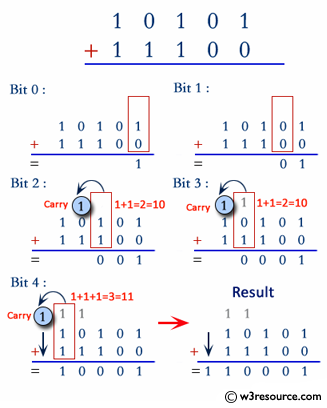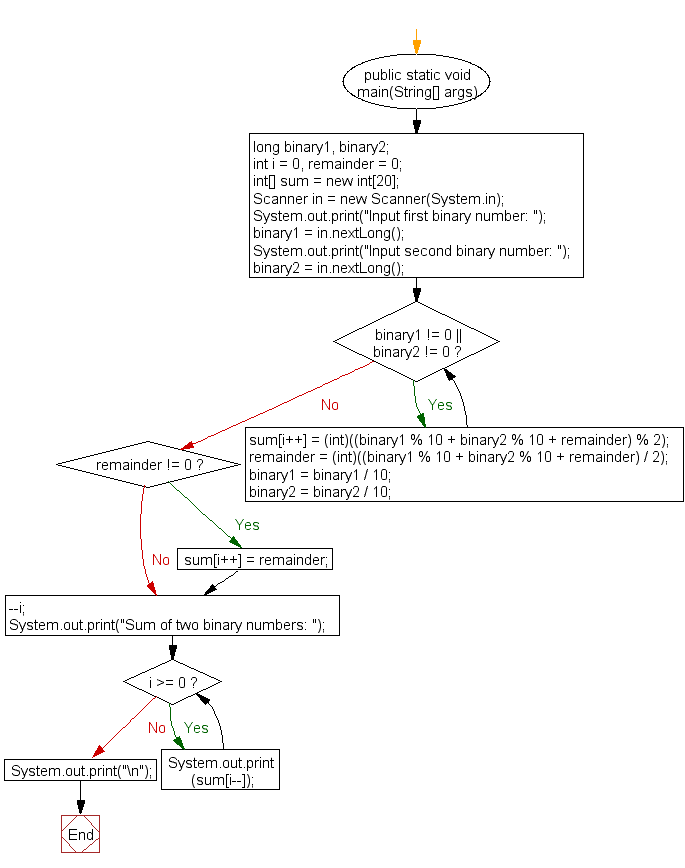﻿ Java exercises: Add two binary numbers - w3resource# Java Exercises: Add two binary numbers

## Java Basic: Exercise-17 with Solution

Write a Java program to add two binary numbers.

In digital electronics and mathematics, a binary number is a number expressed in the base-2 numeral system or binary numeral system. This system uses only two symbols: typically 1 (one) and 0 (zero).

Test Data:
Input first binary number: 100010
Input second binary number: 110010

Pictorial Presentation:Sample Solution:

Java Code:

``````import java.util.Scanner;
public class Exercise17 {
public static void main(String[] args)
{
long binary1, binary2;
int i = 0, remainder = 0;
int[] sum = new int;
Scanner in = new Scanner(System.in);

System.out.print("Input first binary number: ");
binary1 = in.nextLong();
System.out.print("Input second binary number: ");
binary2 = in.nextLong();

while (binary1 != 0 || binary2 != 0)
{
sum[i++] = (int)((binary1 % 10 + binary2 % 10 + remainder) % 2);
remainder = (int)((binary1 % 10 + binary2 % 10 + remainder) / 2);
binary1 = binary1 / 10;
binary2 = binary2 / 10;
}
if (remainder != 0) {
sum[i++] = remainder;
}
--i;
System.out.print("Sum of two binary numbers: ");
while (i >= 0) {
System.out.print(sum[i--]);
}
System.out.print("\n");
}
}
```
```

Sample Output:

```Input first binary number: 100010
Input second binary number: 110010
Sum of two binary numbers: 1010100
```

Flowchart:Java Code Editor:

What is the difficulty level of this exercise?

Test your Programming skills with w3resource's quiz.

﻿

## Java: Tips of the Day

countOccurrences

Counts the occurrences of a value in an array.

Use Arrays.stream().filter().count() to count total number of values that equals the specified value.

```public static long countOccurrences(int[] numbers, int value) {
return Arrays.stream(numbers)
.filter(number -> number == value)
.count();
}
```

Ref: https://bit.ly/3kCAgLb